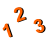You are here: Start » AVL.NET » Regression Analysis

# Regression Analysis

IconNameDescriptionLinearRegressionComputes linear regression of given point set.LinearRegression_LTEComputes linear regression of given point set using Least Trimmed Error algorithm.LinearRegression_MComputes linear regression of given point set using selected M-estimator for outlier suppression.LinearRegression_RANSACComputes linear regression of given point set using RANSAC.LinearRegression_TheilSenComputes linear regression of given point set using TheilSen algorithm.QuadraticRegressionComputes quadratic regression of given point set.QuadraticRegression_MComputes quadratic regression of given point set using selected M-estimator for outlier suppression.QuadraticRegression_RANSACComputes quadratic regression of given point set using RANSAC.Statistics_OfArrayComputes basic statistical information out of an array of real numbers. The array must be not empty.Statistics_OfLoopComputes basic statistical information out of real numbers appearing in consecutive iterations.